Скачать презентацию Amplitude Modulation AM Learning Objectives At the end

7f6bb7babc889a173d51a8371e416e32.ppt

• Количество слайдов: 33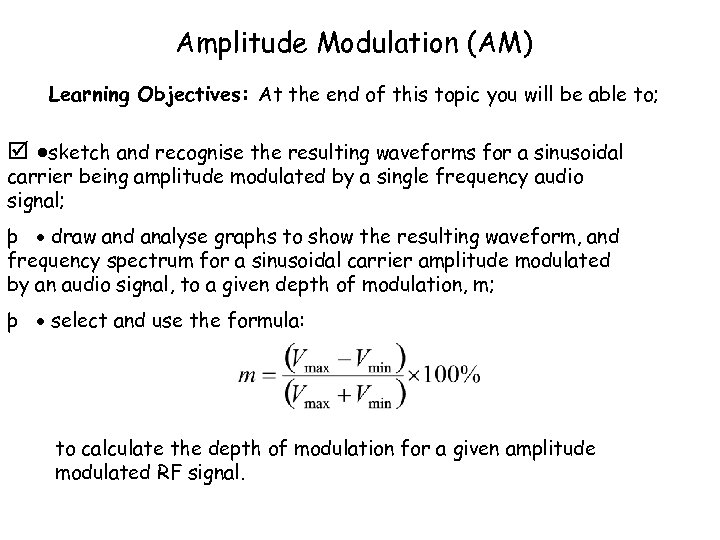Amplitude Modulation (AM) Learning Objectives: At the end of this topic you will be able to; þ sketch and recognise the resulting waveforms for a sinusoidal carrier being amplitude modulated by a single frequency audio signal; þ draw and analyse graphs to show the resulting waveform, and frequency spectrum for a sinusoidal carrier amplitude modulated by an audio signal, to a given depth of modulation, m; þ select and use the formula: to calculate the depth of modulation for a given amplitude modulated RF signal.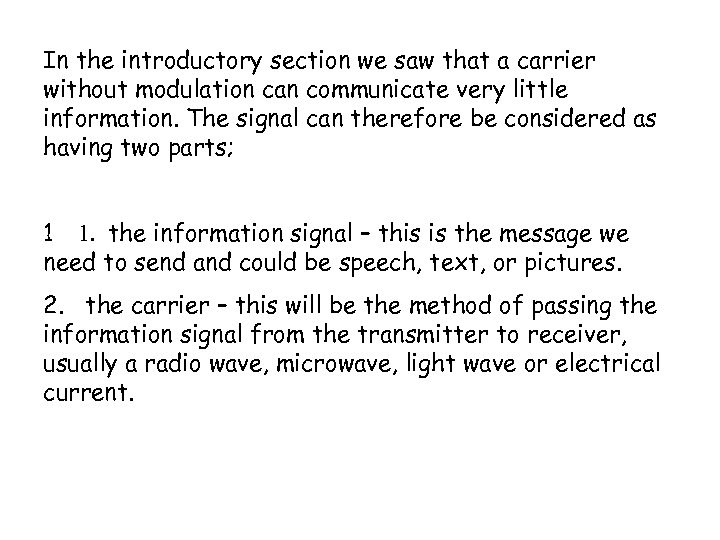In the introductory section we saw that a carrier without modulation can communicate very little information. The signal can therefore be considered as having two parts; 1 1. the information signal – this is the message we need to send and could be speech, text, or pictures. 2. the carrier – this will be the method of passing the information signal from the transmitter to receiver, usually a radio wave, microwave, light wave or electrical current.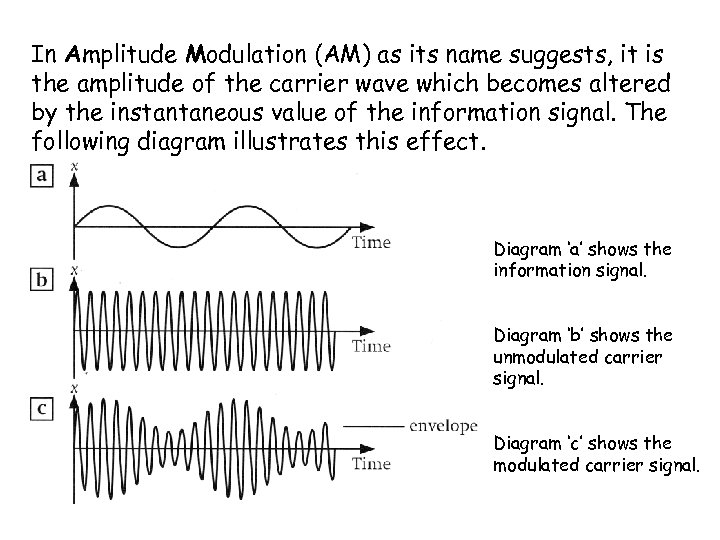In Amplitude Modulation (AM) as its name suggests, it is the amplitude of the carrier wave which becomes altered by the instantaneous value of the information signal. The following diagram illustrates this effect. Diagram ‘a’ shows the information signal. Diagram ‘b’ shows the unmodulated carrier signal. Diagram ‘c’ shows the modulated carrier signal.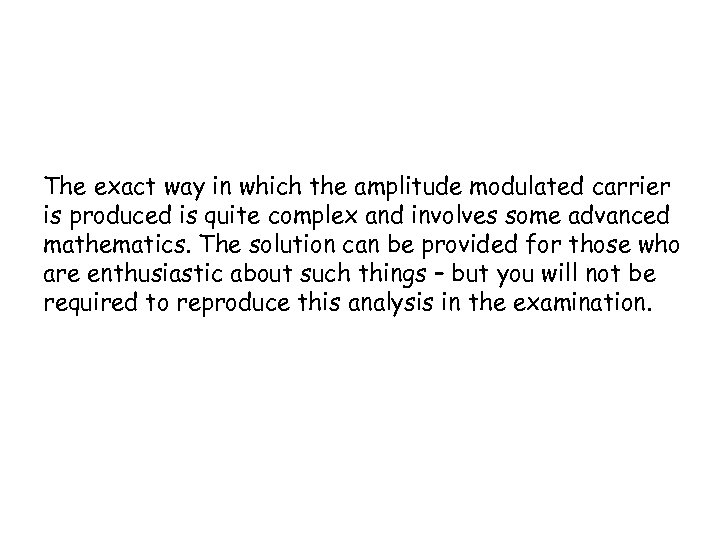The exact way in which the amplitude modulated carrier is produced is quite complex and involves some advanced mathematics. The solution can be provided for those who are enthusiastic about such things – but you will not be required to reproduce this analysis in the examination.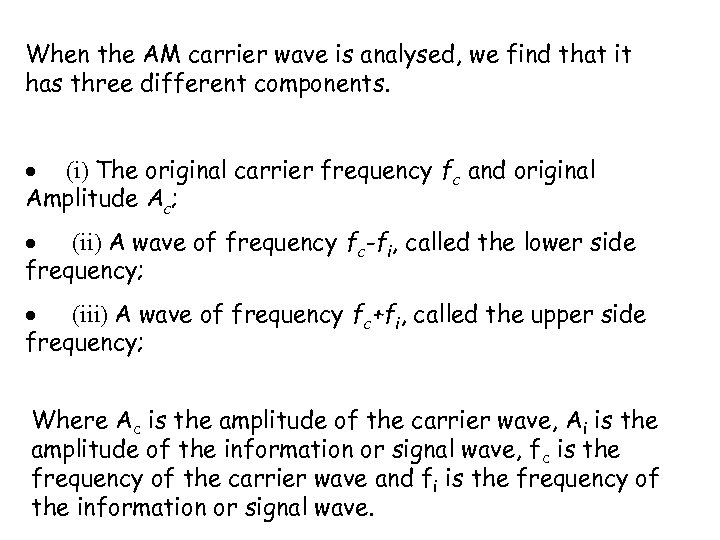When the AM carrier wave is analysed, we find that it has three different components. (i) The original carrier frequency fc and original Amplitude Ac; (ii) A wave of frequency fc-fi, called the lower side frequency; (iii) A wave of frequency fc+fi, called the upper side frequency; Where Ac is the amplitude of the carrier wave, Ai is the amplitude of the information or signal wave, fc is the frequency of the carrier wave and fi is the frequency of the information or signal wave.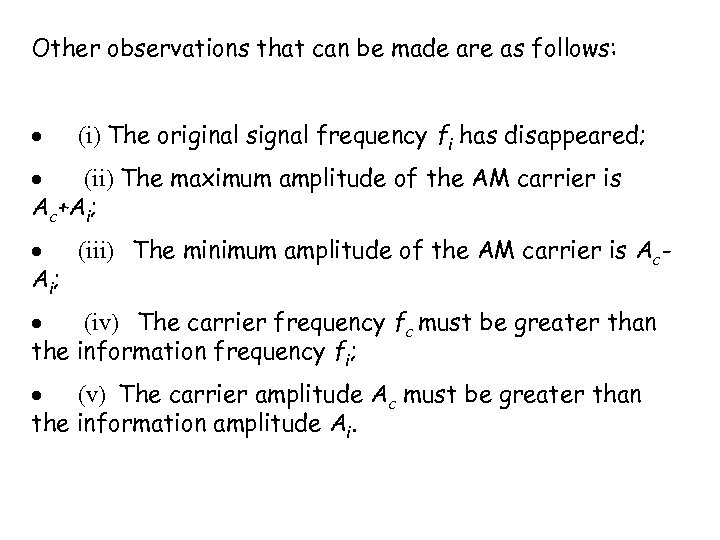Other observations that can be made are as follows: (i) The original signal frequency fi has disappeared; (ii) The maximum amplitude of the AM carrier is Ac+Ai; (iii) The minimum amplitude of the AM carrier is Ac. A i; (iv) The carrier frequency fc must be greater than the information frequency fi; (v) The carrier amplitude Ac must be greater than the information amplitude Ai.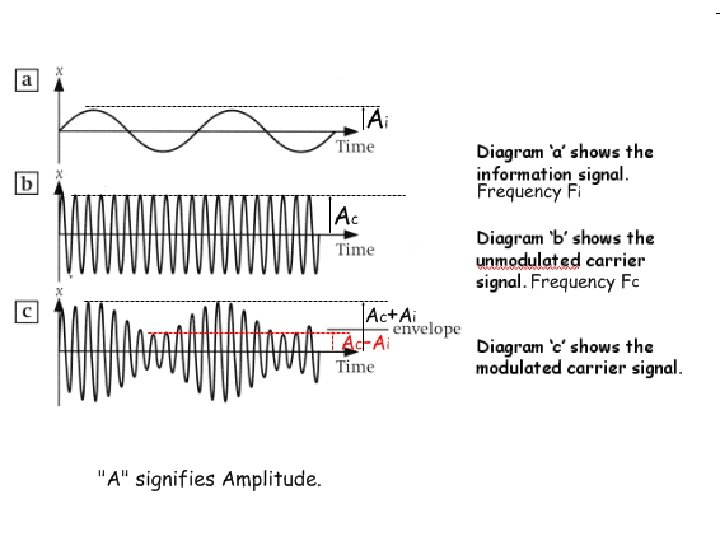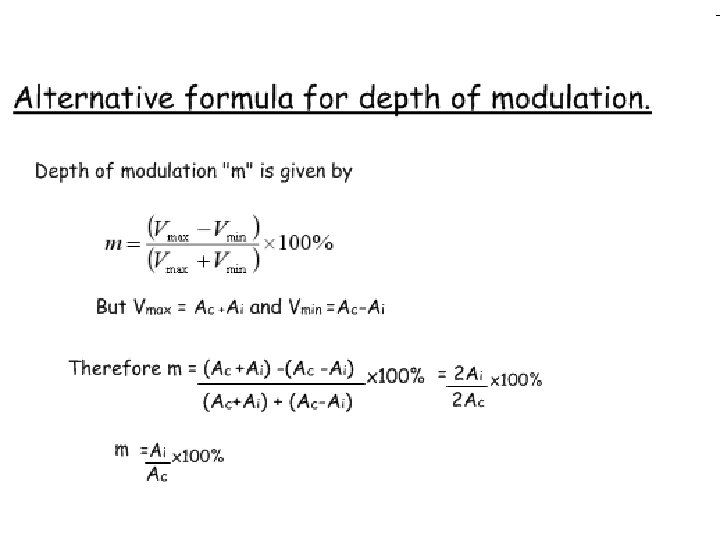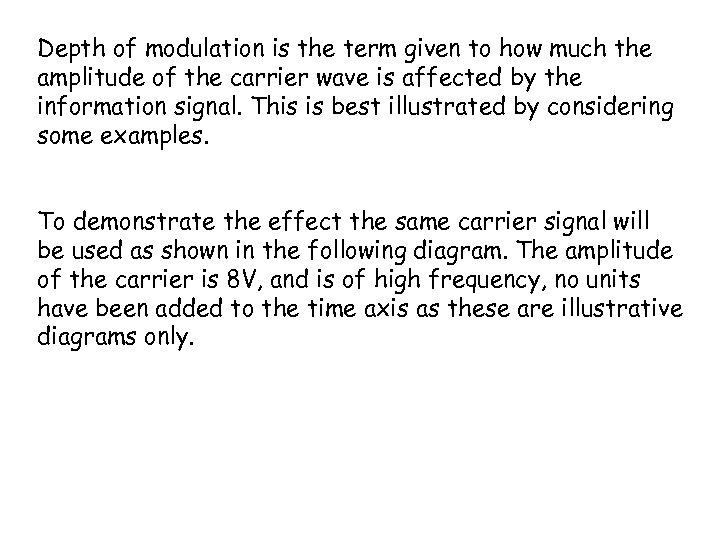Depth of modulation is the term given to how much the amplitude of the carrier wave is affected by the information signal. This is best illustrated by considering some examples. To demonstrate the effect the same carrier signal will be used as shown in the following diagram. The amplitude of the carrier is 8 V, and is of high frequency, no units have been added to the time axis as these are illustrative diagrams only.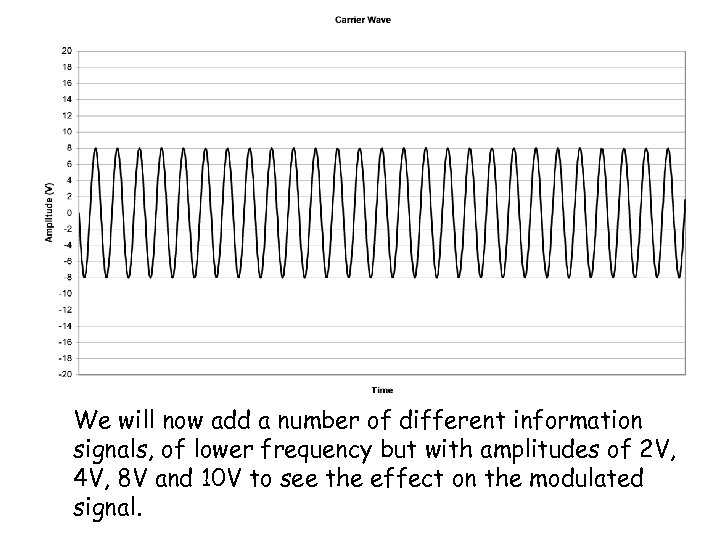We will now add a number of different information signals, of lower frequency but with amplitudes of 2 V, 4 V, 8 V and 10 V to see the effect on the modulated signal.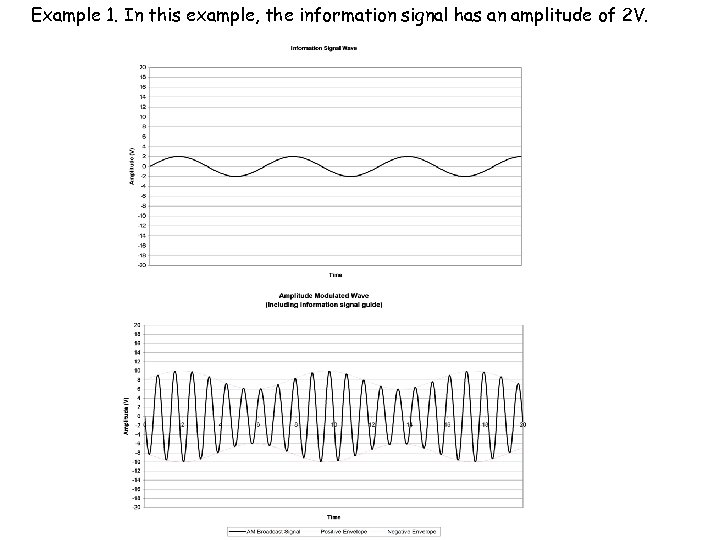Example 1. In this example, the information signal has an amplitude of 2 V.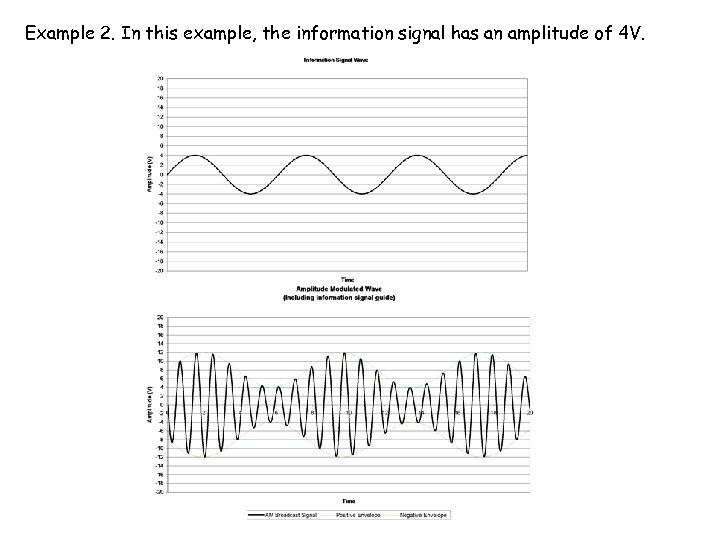Example 2. In this example, the information signal has an amplitude of 4 V.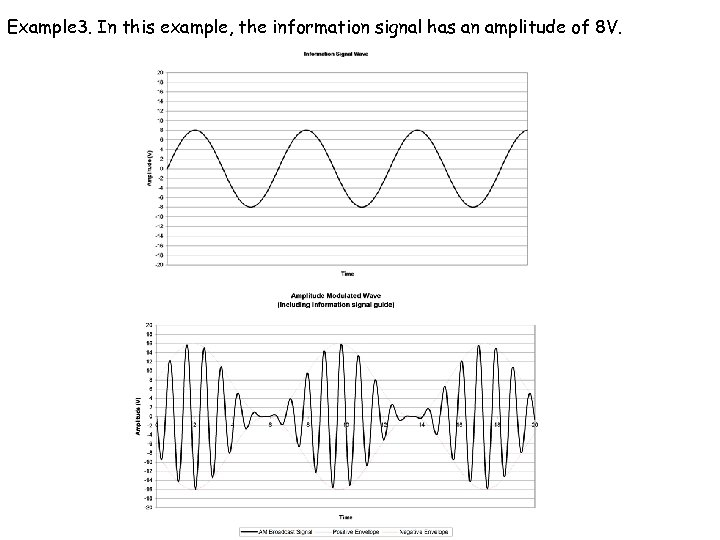Example 3. In this example, the information signal has an amplitude of 8 V.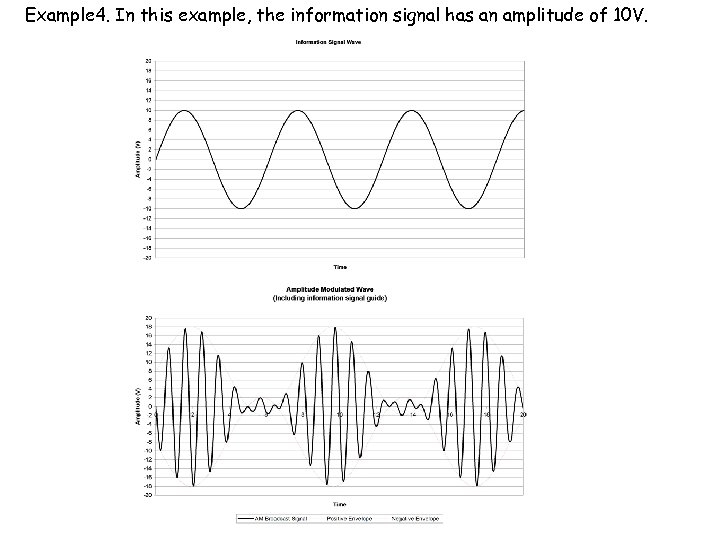Example 4. In this example, the information signal has an amplitude of 10 V.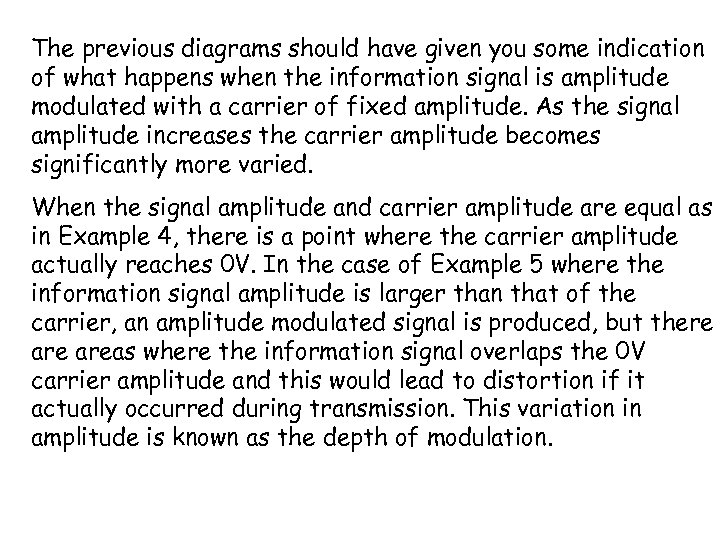The previous diagrams should have given you some indication of what happens when the information signal is amplitude modulated with a carrier of fixed amplitude. As the signal amplitude increases the carrier amplitude becomes significantly more varied. When the signal amplitude and carrier amplitude are equal as in Example 4, there is a point where the carrier amplitude actually reaches 0 V. In the case of Example 5 where the information signal amplitude is larger than that of the carrier, an amplitude modulated signal is produced, but there areas where the information signal overlaps the 0 V carrier amplitude and this would lead to distortion if it actually occurred during transmission. This variation in amplitude is known as the depth of modulation.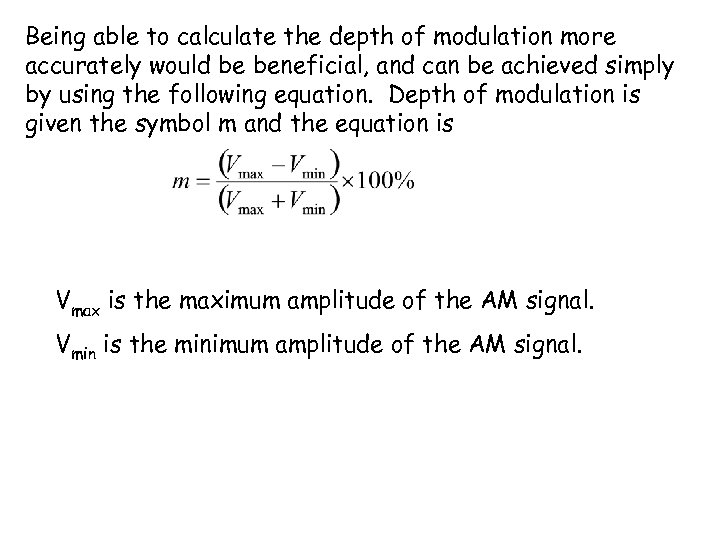Being able to calculate the depth of modulation more accurately would be beneficial, and can be achieved simply by using the following equation. Depth of modulation is given the symbol m and the equation is Vmax is the maximum amplitude of the AM signal. Vmin is the minimum amplitude of the AM signal.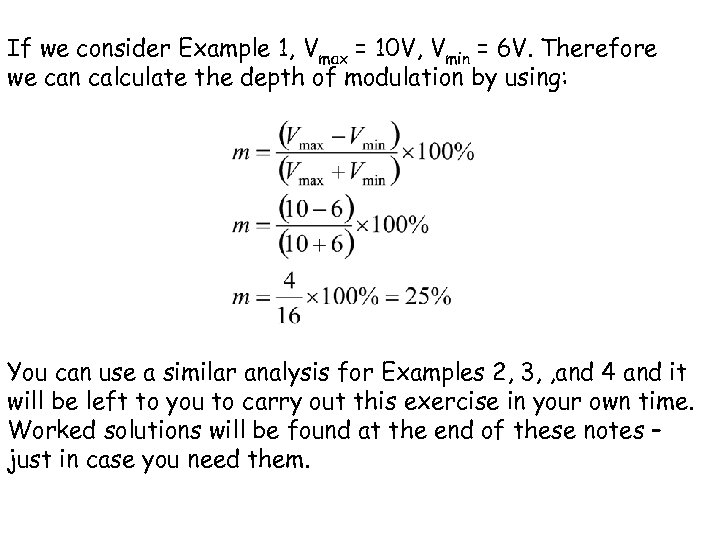If we consider Example 1, Vmax = 10 V, Vmin = 6 V. Therefore we can calculate the depth of modulation by using: You can use a similar analysis for Examples 2, 3, , and 4 and it will be left to you to carry out this exercise in your own time. Worked solutions will be found at the end of these notes – just in case you need them.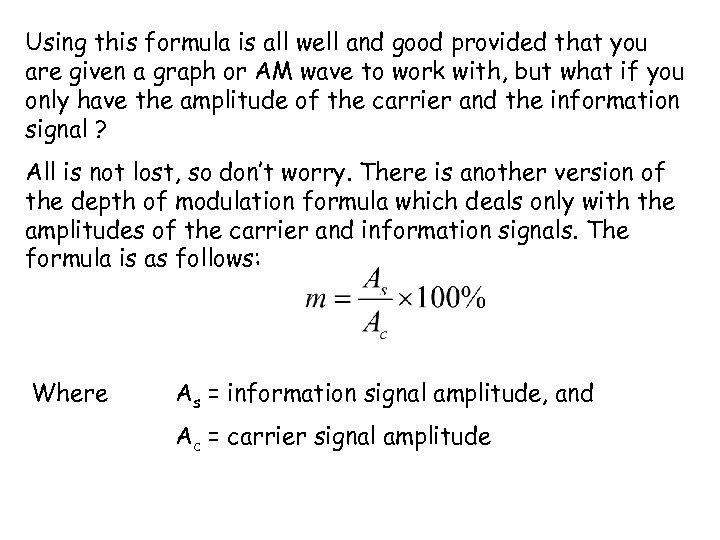Using this formula is all well and good provided that you are given a graph or AM wave to work with, but what if you only have the amplitude of the carrier and the information signal ? All is not lost, so don’t worry. There is another version of the depth of modulation formula which deals only with the amplitudes of the carrier and information signals. The formula is as follows: Where As = information signal amplitude, and Ac = carrier signal amplitude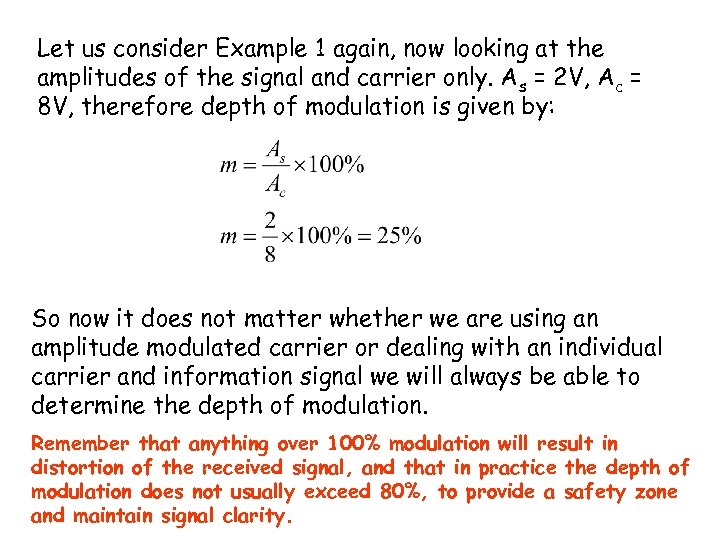Let us consider Example 1 again, now looking at the amplitudes of the signal and carrier only. As = 2 V, Ac = 8 V, therefore depth of modulation is given by: So now it does not matter whether we are using an amplitude modulated carrier or dealing with an individual carrier and information signal we will always be able to determine the depth of modulation. Remember that anything over 100% modulation will result in distortion of the received signal, and that in practice the depth of modulation does not usually exceed 80%, to provide a safety zone and maintain signal clarity.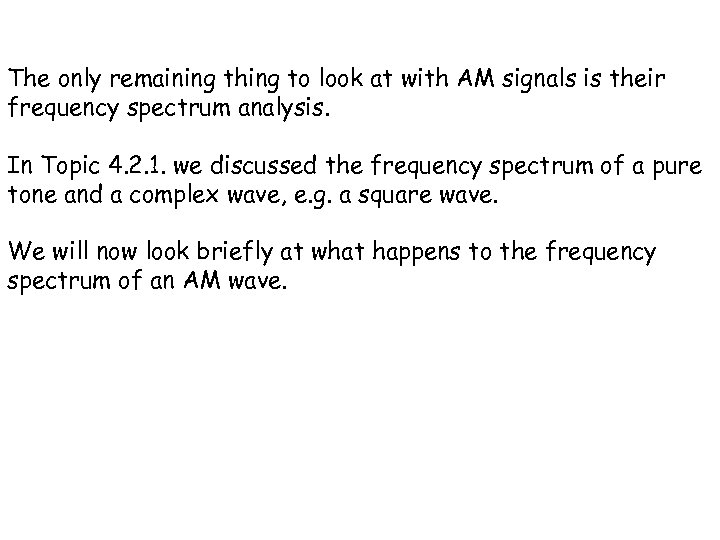The only remaining thing to look at with AM signals is their frequency spectrum analysis. In Topic 4. 2. 1. we discussed the frequency spectrum of a pure tone and a complex wave, e. g. a square wave. We will now look briefly at what happens to the frequency spectrum of an AM wave.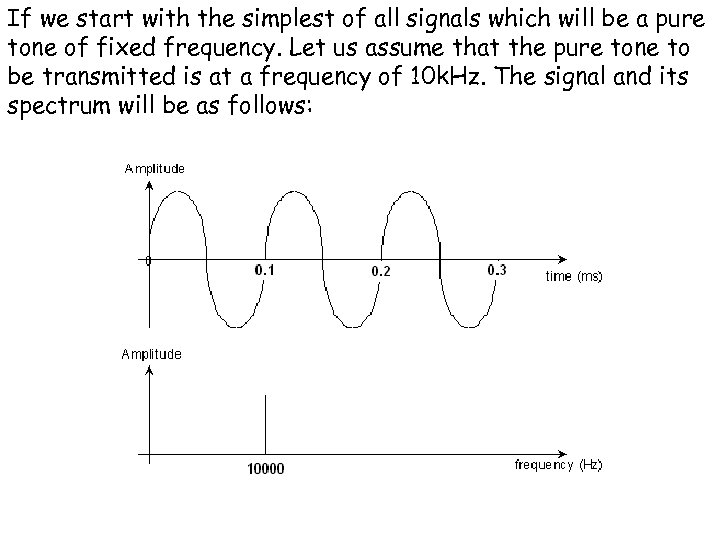If we start with the simplest of all signals which will be a pure tone of fixed frequency. Let us assume that the pure tone to be transmitted is at a frequency of 10 k. Hz. The signal and its spectrum will be as follows: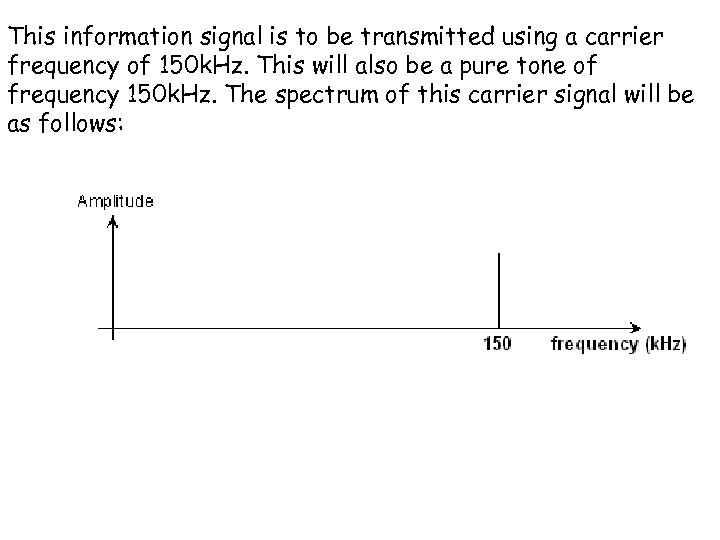This information signal is to be transmitted using a carrier frequency of 150 k. Hz. This will also be a pure tone of frequency 150 k. Hz. The spectrum of this carrier signal will be as follows: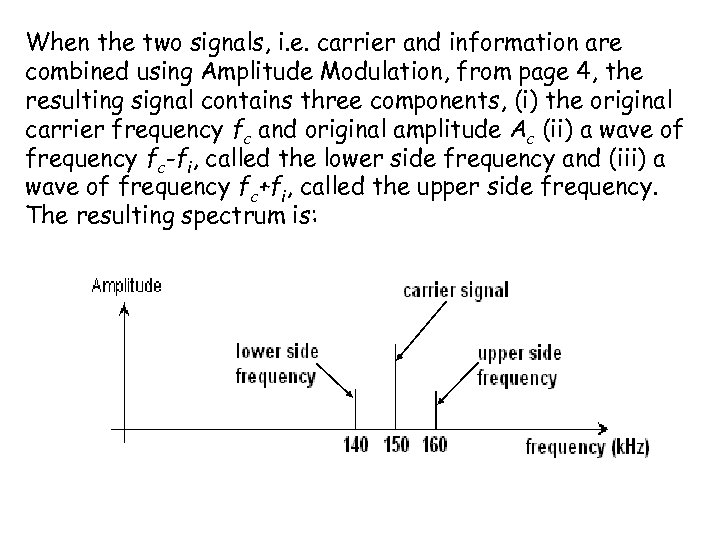When the two signals, i. e. carrier and information are combined using Amplitude Modulation, from page 4, the resulting signal contains three components, (i) the original carrier frequency fc and original amplitude Ac (ii) a wave of frequency fc-fi, called the lower side frequency and (iii) a wave of frequency fc+fi, called the upper side frequency. The resulting spectrum is: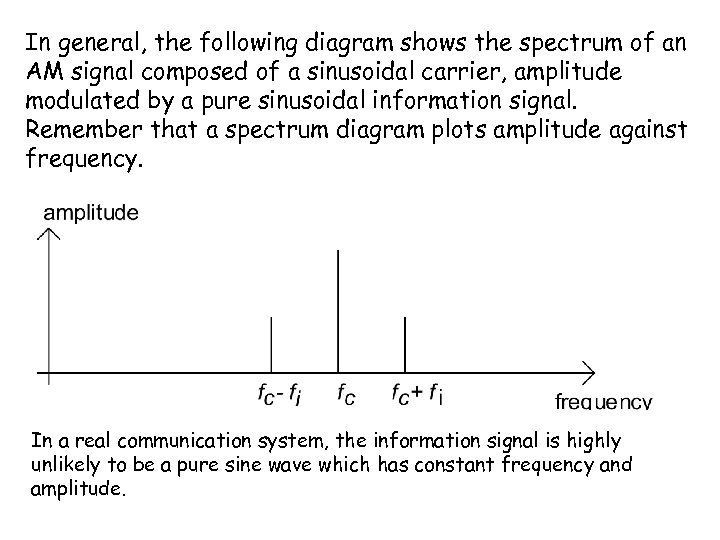In general, the following diagram shows the spectrum of an AM signal composed of a sinusoidal carrier, amplitude modulated by a pure sinusoidal information signal. Remember that a spectrum diagram plots amplitude against frequency. In a real communication system, the information signal is highly unlikely to be a pure sine wave which has constant frequency and amplitude.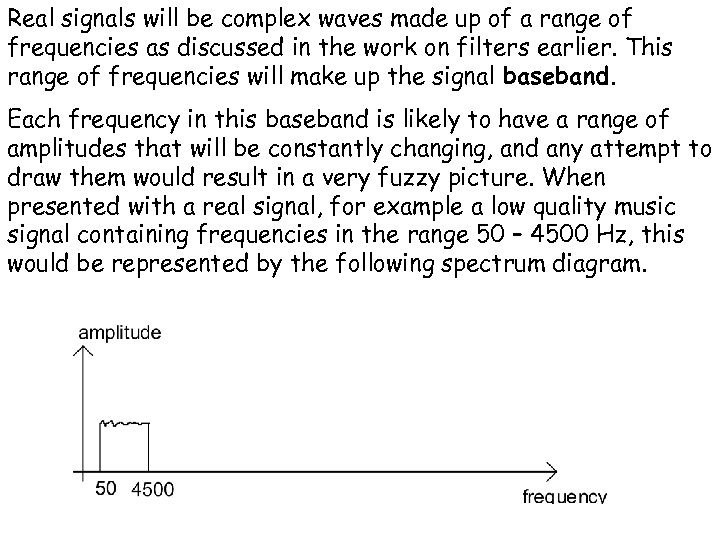Real signals will be complex waves made up of a range of frequencies as discussed in the work on filters earlier. This range of frequencies will make up the signal baseband. Each frequency in this baseband is likely to have a range of amplitudes that will be constantly changing, and any attempt to draw them would result in a very fuzzy picture. When presented with a real signal, for example a low quality music signal containing frequencies in the range 50 – 4500 Hz, this would be represented by the following spectrum diagram.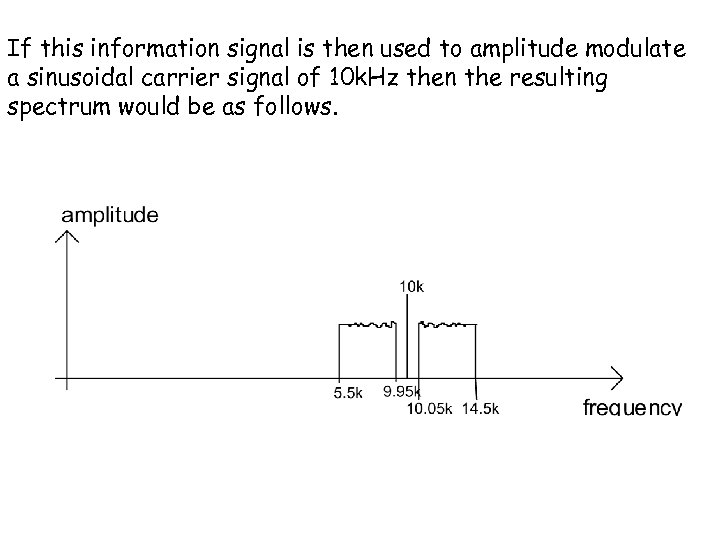If this information signal is then used to amplitude modulate a sinusoidal carrier signal of 10 k. Hz then the resulting spectrum would be as follows.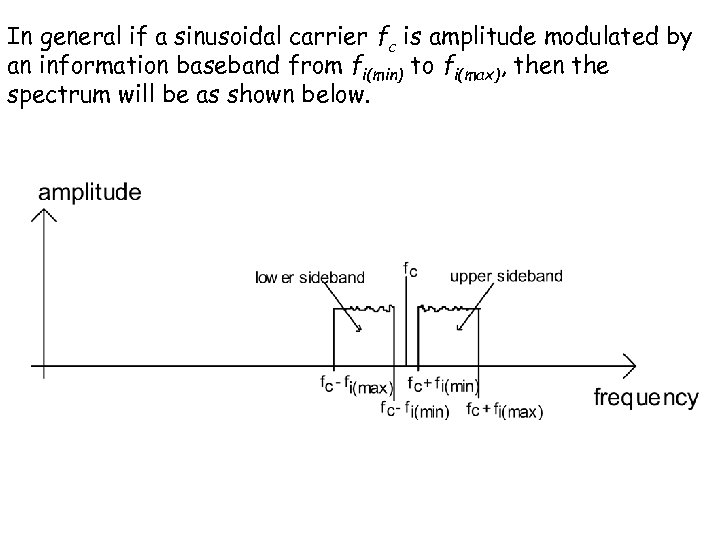In general if a sinusoidal carrier fc is amplitude modulated by an information baseband from fi(min) to fi(max), then the spectrum will be as shown below.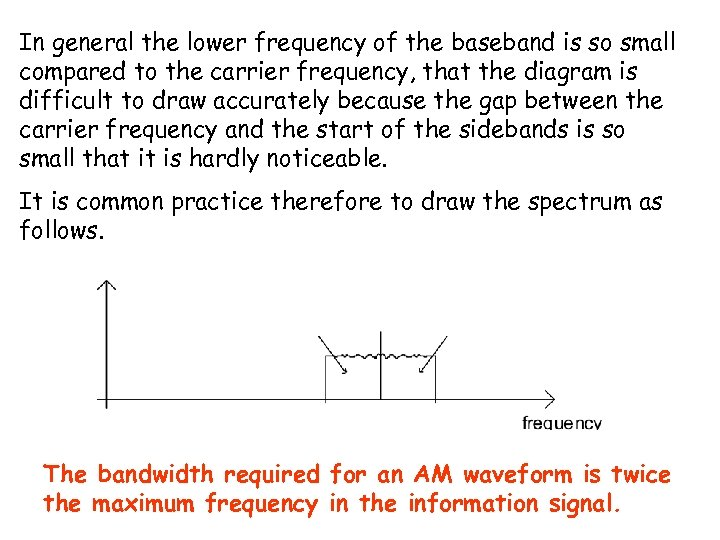In general the lower frequency of the baseband is so small compared to the carrier frequency, that the diagram is difficult to draw accurately because the gap between the carrier frequency and the start of the sidebands is so small that it is hardly noticeable. It is common practice therefore to draw the spectrum as follows. The bandwidth required for an AM waveform is twice the maximum frequency in the information signal.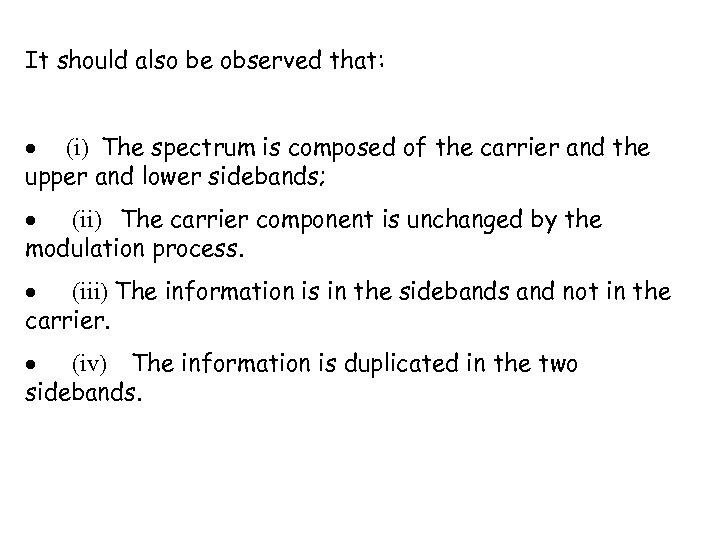It should also be observed that: (i) The spectrum is composed of the carrier and the upper and lower sidebands; (ii) The carrier component is unchanged by the modulation process. (iii) The information is in the sidebands and not in the carrier. (iv) The information is duplicated in the two sidebands.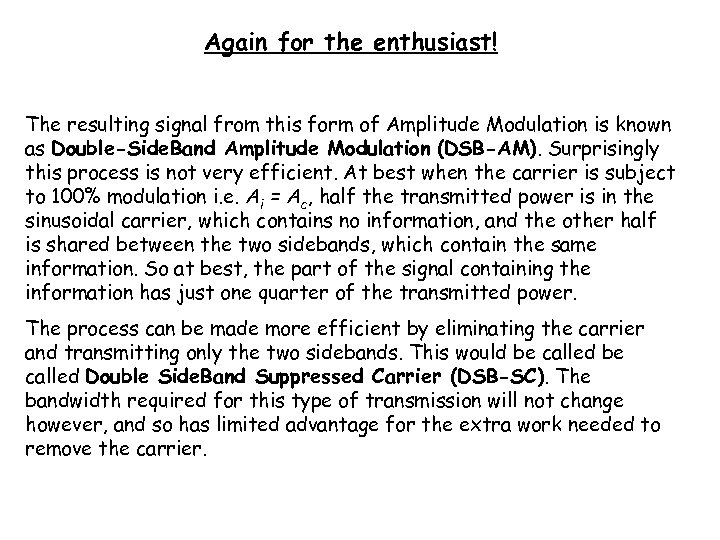Again for the enthusiast! The resulting signal from this form of Amplitude Modulation is known as Double-Side. Band Amplitude Modulation (DSB-AM). Surprisingly this process is not very efficient. At best when the carrier is subject to 100% modulation i. e. Ai = Ac, half the transmitted power is in the sinusoidal carrier, which contains no information, and the other half is shared between the two sidebands, which contain the same information. So at best, the part of the signal containing the information has just one quarter of the transmitted power. The process can be made more efficient by eliminating the carrier and transmitting only the two sidebands. This would be called Double Side. Band Suppressed Carrier (DSB-SC). The bandwidth required for this type of transmission will not change however, and so has limited advantage for the extra work needed to remove the carrier.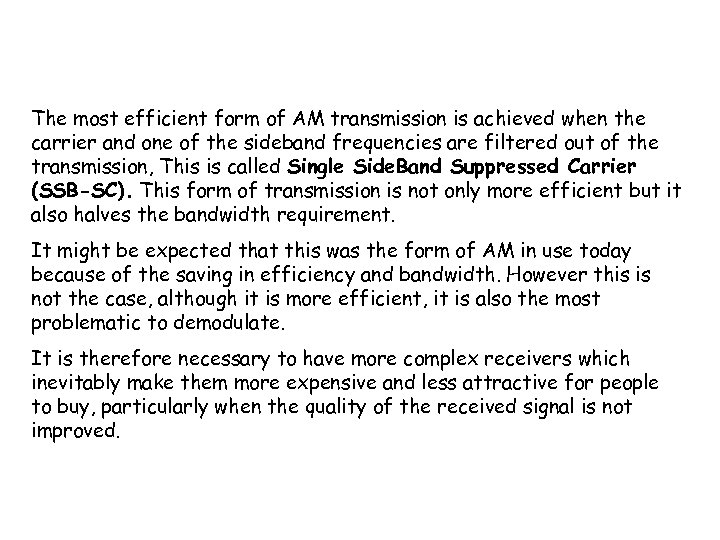The most efficient form of AM transmission is achieved when the carrier and one of the sideband frequencies are filtered out of the transmission, This is called Single Side. Band Suppressed Carrier (SSB-SC). This form of transmission is not only more efficient but it also halves the bandwidth requirement. It might be expected that this was the form of AM in use today because of the saving in efficiency and bandwidth. However this is not the case, although it is more efficient, it is also the most problematic to demodulate. It is therefore necessary to have more complex receivers which inevitably make them more expensive and less attractive for people to buy, particularly when the quality of the received signal is not improved.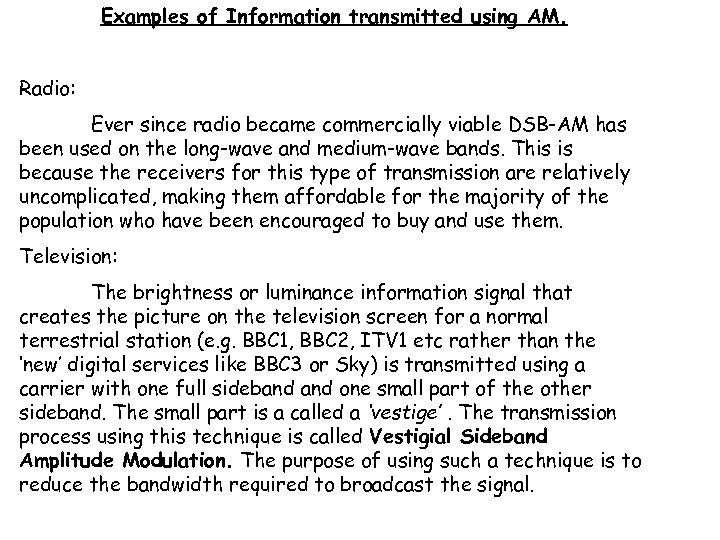Examples of Information transmitted using AM. Radio: Ever since radio became commercially viable DSB-AM has been used on the long-wave and medium-wave bands. This is because the receivers for this type of transmission are relatively uncomplicated, making them affordable for the majority of the population who have been encouraged to buy and use them. Television: The brightness or luminance information signal that creates the picture on the television screen for a normal terrestrial station (e. g. BBC 1, BBC 2, ITV 1 etc rather than the ‘new’ digital services like BBC 3 or Sky) is transmitted using a carrier with one full sideband one small part of the other sideband. The small part is a called a ‘vestige’. The transmission process using this technique is called Vestigial Sideband Amplitude Modulation. The purpose of using such a technique is to reduce the bandwidth required to broadcast the signal.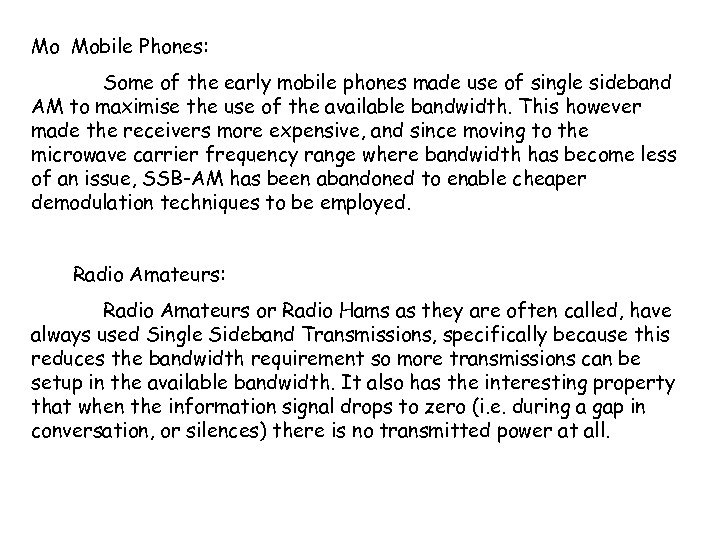Mo Mobile Phones: Some of the early mobile phones made use of single sideband AM to maximise the use of the available bandwidth. This however made the receivers more expensive, and since moving to the microwave carrier frequency range where bandwidth has become less of an issue, SSB-AM has been abandoned to enable cheaper demodulation techniques to be employed. Radio Amateurs: Radio Amateurs or Radio Hams as they are often called, have always used Single Sideband Transmissions, specifically because this reduces the bandwidth requirement so more transmissions can be setup in the available bandwidth. It also has the interesting property that when the information signal drops to zero (i. e. during a gap in conversation, or silences) there is no transmitted power at all.